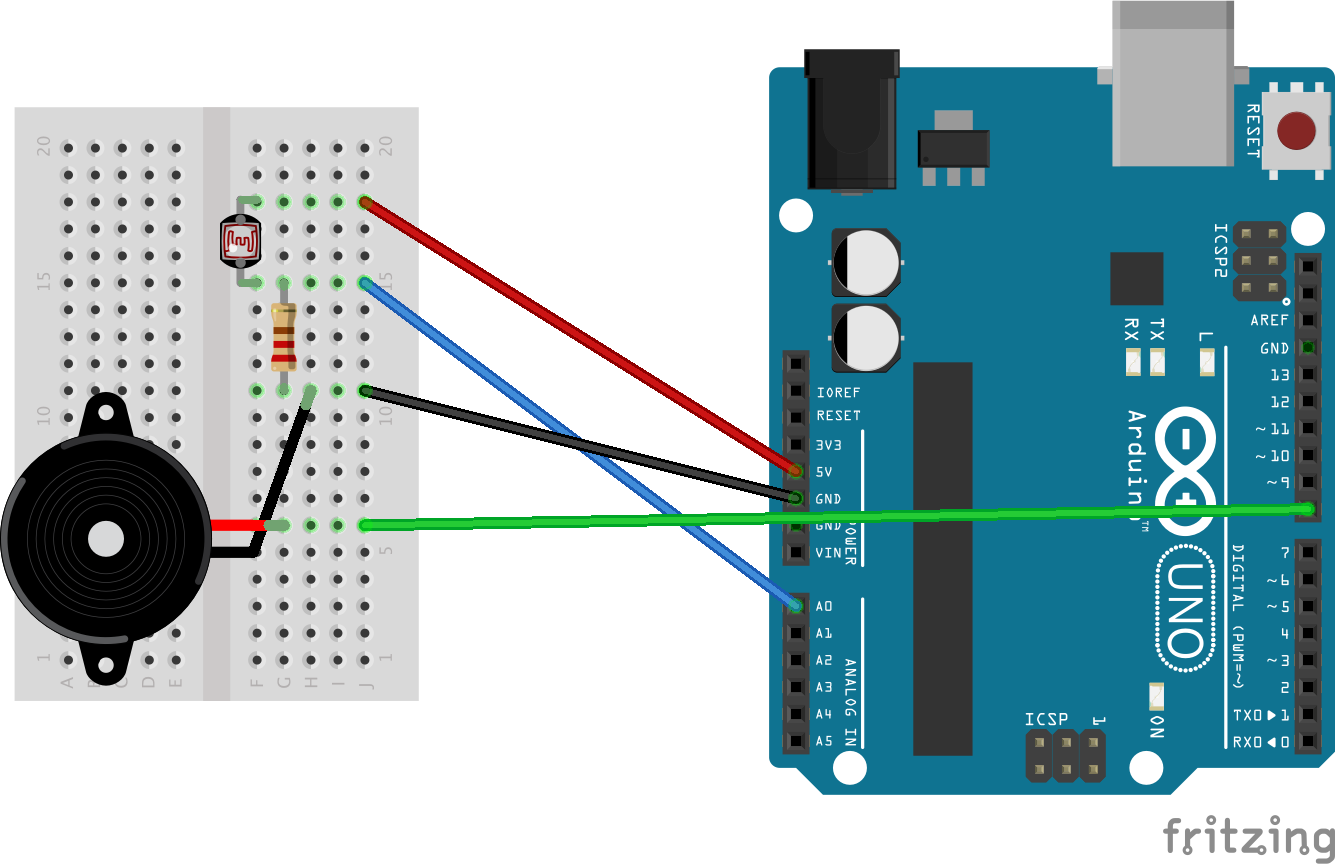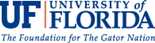# Arduinos: Pseudo-Theremin

Physical Computing, the Internet of Things, Sensors and Servos

## Goals

Goals

• Learn how to use analogRead() function to detect amount of light from photocell
• Learn about translating notes to frequency in Hertz
• Learn how to use tone() to create simple sounds out of piezo
• Learn about the theremin and replicate this instrument

## Arduino Code

/*
*/

int piezo = 8;
int photocell = A0;

void setup()
{
}

void loop()
{
int frequency = 200 + lightInput / 4;
tone(piezo, frequency);
}

## Hardware schematicsIn this set up, imagine that we have two circuits. Both start and end at the Arduino. In the first, we take a wire from 5V to the breadboard to provide power. We then use the same row to connect our photocell to send current through it. The second leg of the photocell goes on a separate row. The current then runs from the second leg of the photocell to A0 to send the analog data being collected from the photocell back to the Arduino. The same row needs one leg of the resistor (bidirectional) and the resistor needs to bridge the breadboard to another row that can be grounded. The grounding wire goes from that row on the breadboard back to the Arduino completing circuit (circle) 1. The Arduino now has the data it needs to determine how much light is shining. So, for output, we take a wire from the Arduino digital pin 8 to a new row on the breadboard. This provides data and current. On this same row is the red wire of the piezo. The black wire of the piezo should be grounded in the same row as the resistor. (There is more than one correct way to build a circuit. You could separately ground each circuit.)

## Electronic Components

"The sketch is actually really straightforward. We simply take an analog reading from A0, to measure the light intensity. This value will be in the range of something like 0 to 700.

We add 200 to this raw value, to make 200 Hz the lowest frequency and simply add the reading divided by 4 to this value, to give us a range of around 200Hz to 370Hz." (https://learn.adafruit.com/adafruit-arduino-lesson-10-making-sounds/arduino-code)